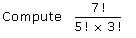Courses

# Test: Permutations Distinct Objects

## 10 Questions MCQ Test Mathematics (Maths) Class 11 | Test: Permutations Distinct Objects

Description
This mock test of Test: Permutations Distinct Objects for JEE helps you for every JEE entrance exam. This contains 10 Multiple Choice Questions for JEE Test: Permutations Distinct Objects (mcq) to study with solutions a complete question bank. The solved questions answers in this Test: Permutations Distinct Objects quiz give you a good mix of easy questions and tough questions. JEE students definitely take this Test: Permutations Distinct Objects exercise for a better result in the exam. You can find other Test: Permutations Distinct Objects extra questions, long questions & short questions for JEE on EduRev as well by searching above.
QUESTION: 1

### In how many ways 4 boys and 3 girls can be seated in a row so that they are alternate.

Solution:

Let the Arrangement be,
B G B G B G B
4 boys can be seated in 4! Ways.
Girl can be seated in 3! Ways.
Required number of ways,
= 4!*3! = 144.

QUESTION: 2

### In how many ways 2 directors and 3 executives can be arranged for a meeting? If there are 6 chairs available two on one side and remaining four on the other side of the table and the two directors has to be together on one side and the executives on the other side.

Solution:

3 executives in 4 chairs = 4P3 ways
Total no. of ways = 2! * 4P3
=> 2*1*(4!/1!)
= 2*24
= 48

QUESTION: 3

### A lady arranges a dinner party for 6 guests .The number of ways in which they may be selected from among 10 friends if 2 of the friends will not attend the party together is

Solution:

Let the friends be A,B,C,D,E,F,G,H,I,J and assume A and B will not battend together
Case 1 : Both of them will not attend the party : Now we have to select the 6 guests from the remaing 8 members
Then no of ways is 8C6 = 28 ways.
Case 2 : Either of them are selected for the party :Now we have to select the 5 guests from the remaing 8 members and  one from A and B
Then the no of ways = 2C1 x 8C5 =112 ways.
Therefore total number of ways is 28+ 112 = 140 ways,

QUESTION: 4

What is the value of npo

Solution:

nP0
= n!/(n-0)!
=> n!/n!  = 1

QUESTION: 5

Find the value of n if nP1 = 10

Solution:

nPr = 10
nPr = n!/(n-r)!
10 = n!/(n-1)!
10 = [n!.(n-1)!]/(n-1)!
n! = 10

QUESTION: 6

Number of signals that can be made using 2 flags out of given 4 flags.

Solution:

No. of ways of selecting two flags out of four = 4C2
So, total possible different signals generated =  4C2×2!
⟹ 6×2=12

QUESTION: 7

What is the value of npn

Solution:

nPn
= n!/(n-n)!
= n!

QUESTION: 8Solution: 7! /5! ×3!
=7×6×5! /5! ×3×2×1
=7
QUESTION: 9

5 singers are to be given performance in a concert. How many ways can they be arranged in a order

Solution:

5P5  ⇒ 5!/(5-5)!
= 5!/0!
= 5!/1 ⇒ 5!
= 120 ways

QUESTION: 10

What is the value of 0!

Solution:

The value of 0! = 1